# The SEQDESIGN Procedure

Subsections:

The Whitehead methods (Whitehead and Stratton 1983; Whitehead 1997, 2001) derive boundary values by adjusting the boundary values generated from continuous monitoring. With continuous monitoring, the boundary values are on a straight line in the score scale for each boundary. For a group sequential design, the boundary values at an interim stage k depend on the information fractions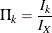where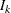is the information available at stage k and
is the maximum information, the information available at the end of the trial if the trial does not stop early.

#### One-Sided Symmetric Designs

A one-sided symmetric design is a one-sided design with identical Type I and Type II error probabilities. For a one-sided symmetric design with an upper alternative,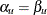, the boundary values in the score scale from continuous monitoring are as follows:

•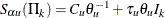•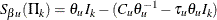where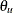is the upper alternative reference,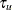is a specified constant for the slope,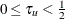, and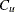is a constant, fixed for STOP=BOTH and derived for STOP=ACCEPT and STOP=REJECT.

The upper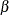boundary value can also be expressed as

•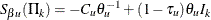Thus, these straight-line boundaries form a triangle in the score statistic scale.

To adjust for the nature of discrete monitoring, the group sequential boundary values are given by the following:

•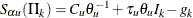•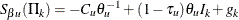where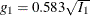and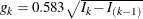,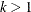are the adjustments.

Note that with the adjustment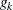, the resulting boundaries form a Christmas tree shape within the original triangle and are referred to as the Christmas tree boundaries (Whitehead 1997, p. 73).

#### One-Sided Asymmetric Designs

For a one-sided asymmetric design with an upper alternative,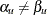, the boundary values computed using the score scale, are given by the following:

•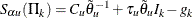•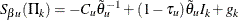where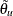is the modified alternative reference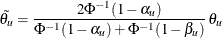The modified alternative reference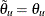if.

For a design with early stopping to reject or accept the null hypothesis,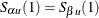, the boundary values at the final stage are equal. The modified drift parameter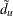is given by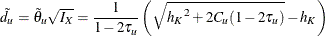where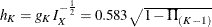.

A one-sided Whitehead design with early stopping to reject or accept the null hypothesis is illustrated in Example 101.7.

#### Two-Sided Designs

The boundary values for a two-sided design are generated by combining boundary values from two one-sided designs. With the STOP=BOTH option, this produces a double triangular design (Whitehead 1997, p. 98).

The boundary values for a two-sided design, using the score scale, are then given by the following:

•••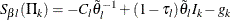•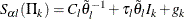where the modified alternative references are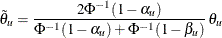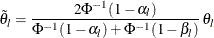The modified alternative referenceifand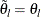if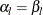.

For a design with early stopping to reject or accept the null hypothesis, the two upper boundary values at the final stage are identical and the two lower boundary values at the final stage are identical. That is,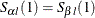and. These modified drift parameters are then given by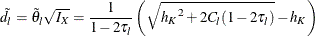where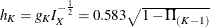.

For a design with early stopping to reject the null hypothesis, or a design with early stopping to accept the null hypothesis, you can specify the slope parametersand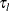in the TAU= option, and then the intercept parametersand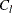, and the resulting boundary values are derived. If both the maximum information and alternative references are specified, the procedure derivesandby maintaining either the overalllevels (BOUNDARYKEY=ALPHA) or the overalllevels (BOUNDARYKEY=BETA). If the maximum information and alternative reference are not both specified, the procedure derives the boundary valuesandby maintaining both the overalland overalllevels.

For a design with early stopping to reject or accept the null hypothesis (STOP=BOTH), Whitehead’s triangular test uses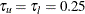and compute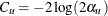and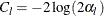for the boundary values. If the maximum information and alternative reference are both specified, the BOUNDARYKEY=ALPHA option uses the specifiedvalues to compute thevalues and boundary values. The final-stage boundary values are modified to maintain the overalllevels if they exist. Similarly, the BOUNDARYKEY=BETA option uses the specifiedvalues to compute thevalues and boundary values. The final-stage boundary values are modified to maintain the overalllevels if they exist.

If the maximum information and alternative reference are not both specified, the specifiedandvalues are used to derive boundary values. The BOUNDARYKEY=NONE option uses these boundary values without adjustment. The BOUNDARYKEY=ALPHA option modifies the final-stage boundary values to maintain the overalllevels if they exist. Similarly, the BOUNDARYKEY=BETA option modifies the final-stage boundary values to maintain the overalllevels if they exist.

#### Applicable Boundary Keys

Table 101.7 lists applicable boundary keys for a design that uses Whitehead methods.

Table 101.7: Applicable Boundary Keys for Whitehead Methods

Specified Parameters

Boundary Keys

Early Stopping

(Alt Ref – Max Info)

Tau

Alpha

Beta

None

Both

Reject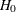X

X

X

X

AcceptX

X

X

X

Reject/AcceptX

0.25

X

X

RejectX

X

AcceptX

X

Reject/Accept0.25

X

X

X

Note that the symbol "X" under "(Alt Ref – Max Info)" indicates that both alternative reference and maximum information are specified.

For a design with early stopping to reject the null hypothesis, or a design with early stopping to accept the null hypothesis, you can specify the slope parameterin the TAU= option, and then the intercept parameterand the resulting boundary values are derived. If both the maximum information and alternative reference are specified, the procedure derivesby maintaining either the overalllevels (BOUNDARYKEY=ALPHA) or the overalllevels (BOUNDARYKEY=BETA). If the maximum information and alternative reference are not both specified, the procedure derives the boundary values andby maintaining both the overalland overalllevels.

For a design with early stopping to reject or accept the null hypothesis (STOP=BOTH), Whitehead’s triangular test uses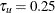and solves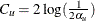for the boundary values. If the maximum information and alternative reference are both specified, the BOUNDARYKEY=ALPHA option uses the specifiedvalue to compute thevalue and boundary values. The final-stage boundary value is modified to maintain the overalllevel if it exists. Similarly, the BOUNDARYKEY=BETA option uses the specifiedvalue to compute thevalue and boundary values. The final-stage boundary value is modified to maintain the overalllevel if it exists.

If the maximum information and alternative reference are not both specified, the specifiedandvalues are used to derive boundary values. The BOUNDARYKEY=NONE option uses these boundary values without adjustment. The BOUNDARYKEY=ALPHA option modifies the final-stage boundary value to maintain the overalllevel if it exists. Similarly, the BOUNDARYKEY=BETA option modifies the final-stage boundary value to maintain the overalllevel if it exists.